# Partitioning 2-digit numbers

## What is partitioning?

Partitioning is the process of breaking down a given number into smaller parts to make it easier to work with. For example, 2-digit numbers can be partitioned into tens and ones.

Although partitioning is strongly linked with place value, it is not the only way to break down a 2-digit number and pupils should be taught to partition numbers in a variety of ways.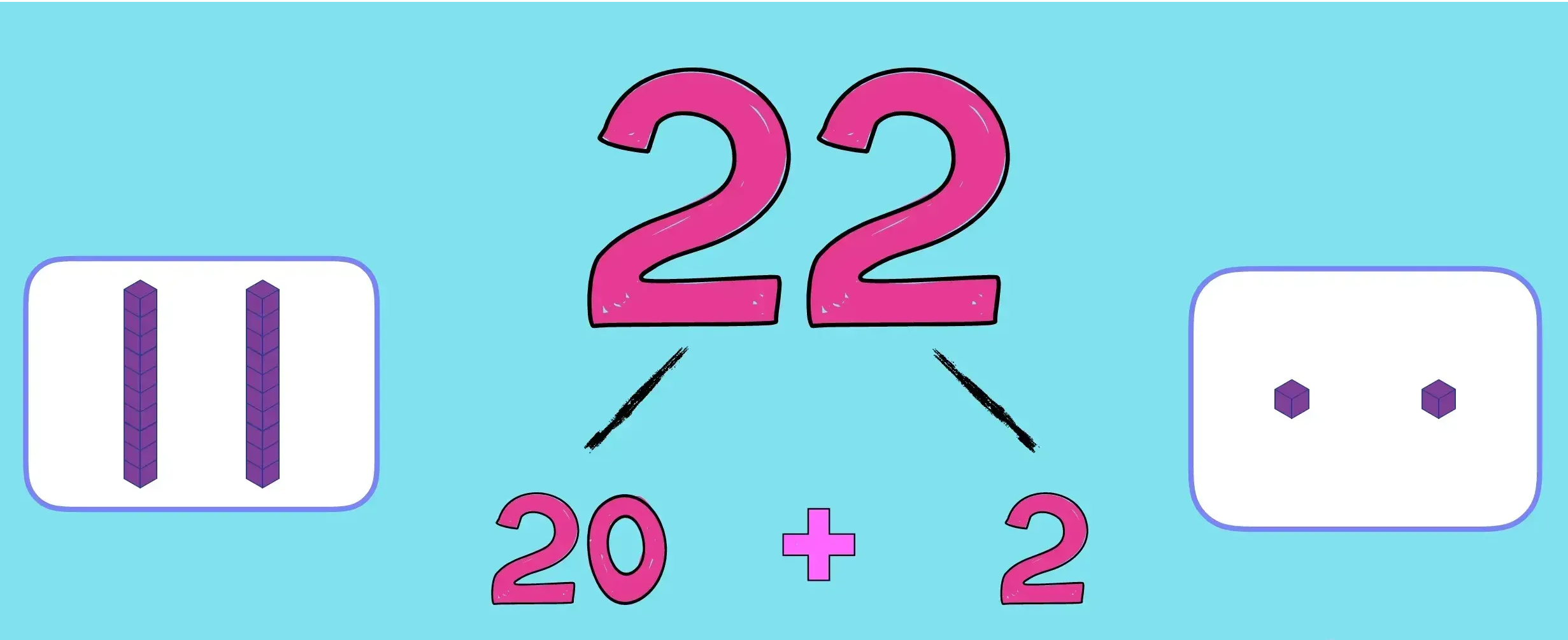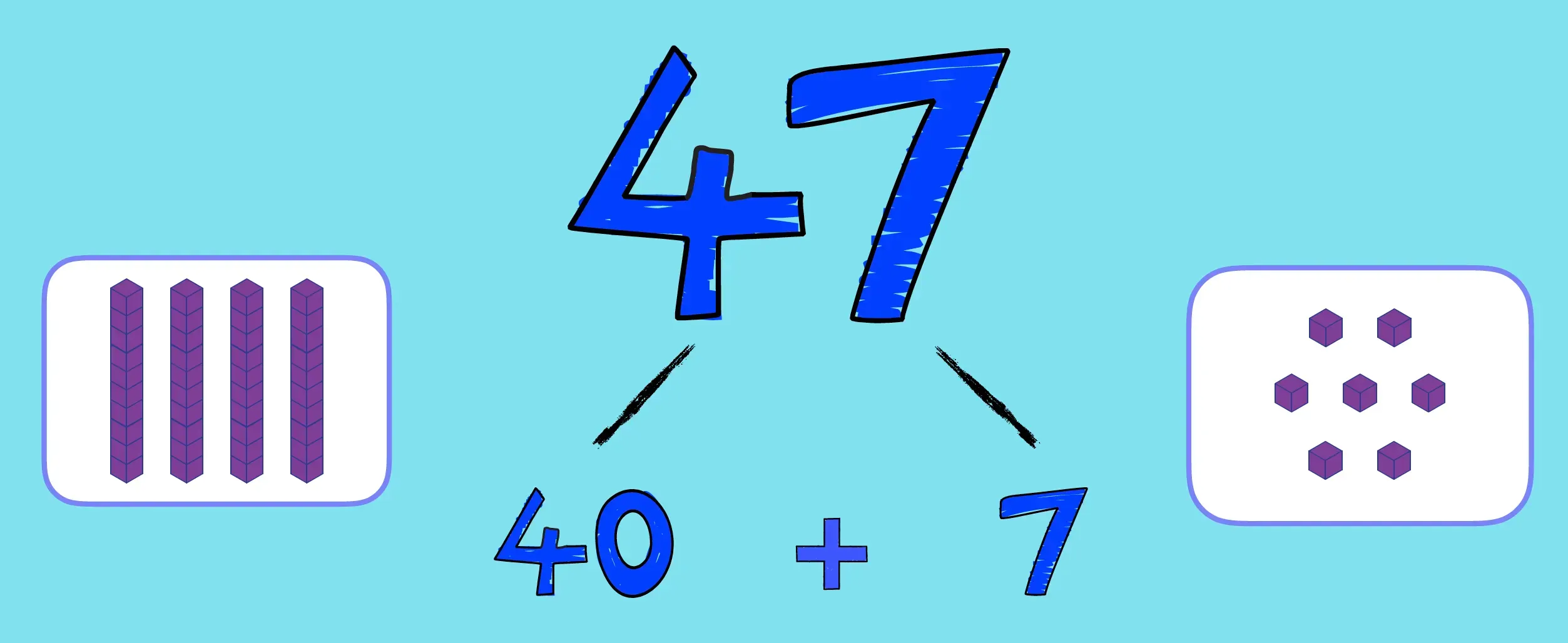## Partitioning in different ways

The skill of partitioning is useful to support children's understanding of number composition, calculation and problem solving.

Partitioning by place value

Children should recognise that the number 64 represents 6 tens and 4 ones. So, the number 64 can be partitioned into 60 and 4 (represented with different models below).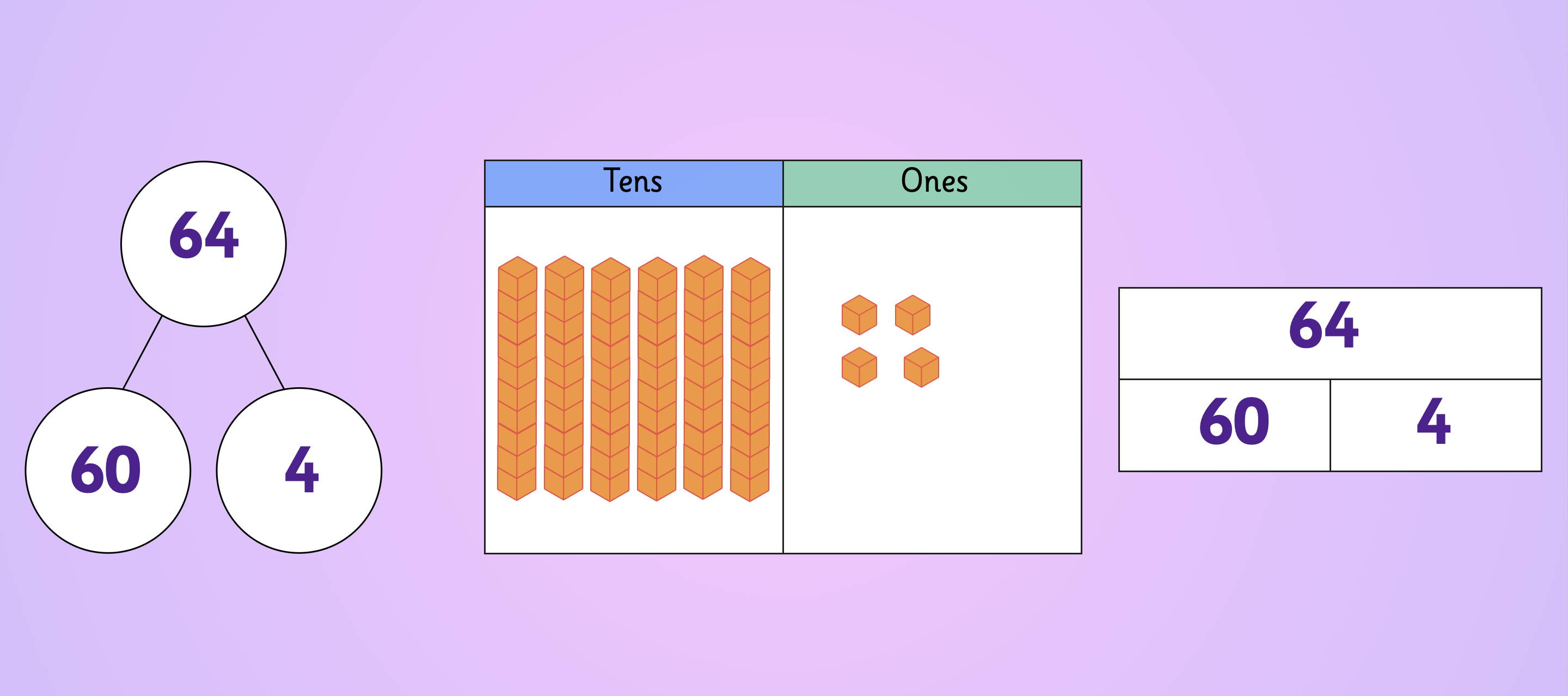## Partitioning to support bridging

Number bonds to ten are another example of partitioning (e.g. 3 + 7 = 10). Children rely on number bonds to bridge ten in their early addition and subtraction work.

Partitioning to support exchange

Partitioning is also called upon in later, formal methods of subtraction. For example, when calculating 364 -18, we must exchange in order to complete the calculation. Exchanging one ten for ten ones requires children to partition the minuend 364 into 300 + 50 + 14.

Partitioning as a fraction

Halving is yet another way to partition numbers. Why not ask children whether it is always possible to halve a 2-digit number for a fun 'always, sometimes, never' investigation.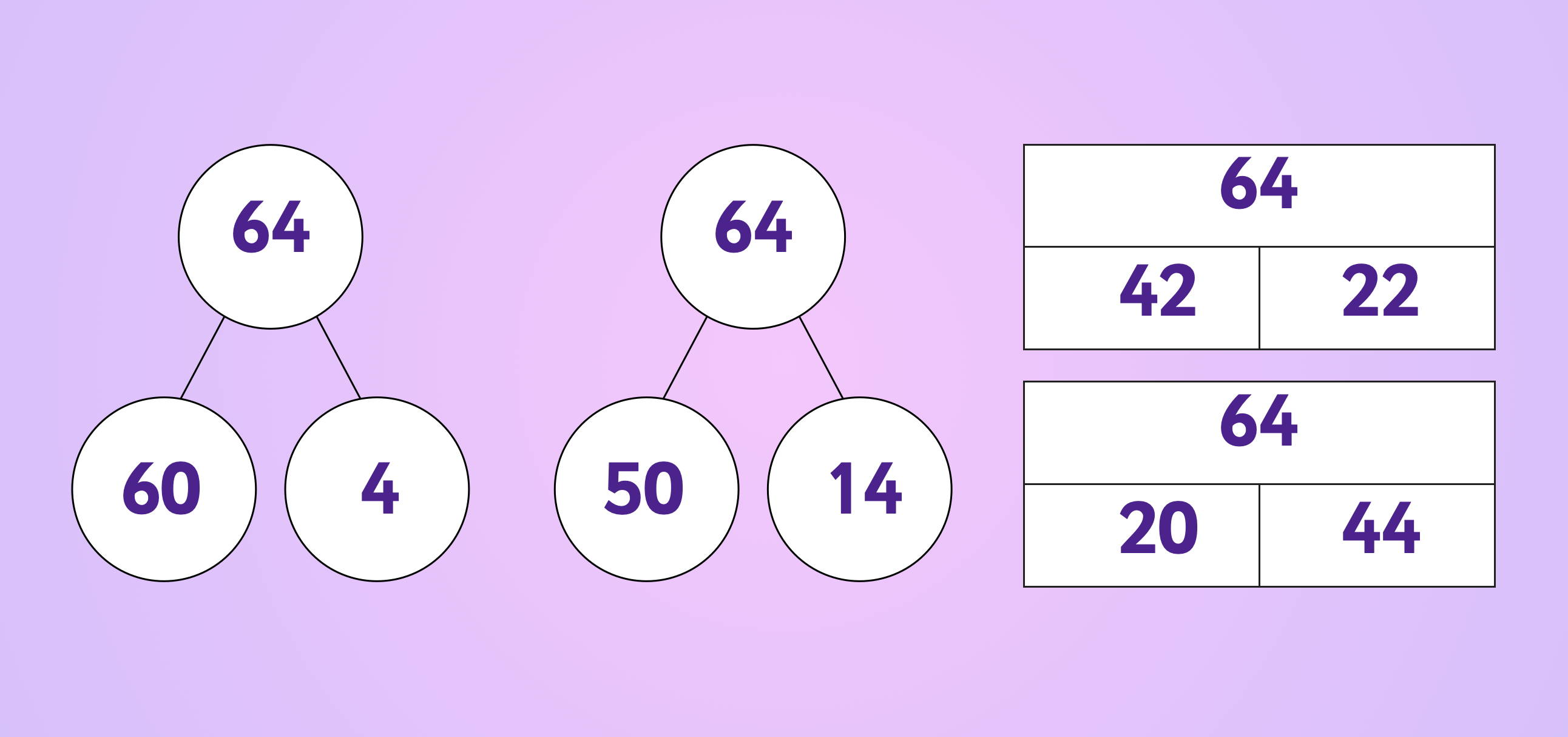## Partitioning games and resources

Partitioning is an area of mathematics that needs a lot of repetition to ensure the concept becomes fully embedded. So, offer children lots of opportunities to practise partitioning numbers using place value charts, part-whole and bar models alongside manipulatives such as numicon, diennes or place value counters.

Make sure to check that children can do not understand partitioning only in terms of place value and that they can partition flexibly, in different ways.

Here are some of our favourite partitioning games:

• For an engaging maths starter, give children a 2-digit number and ask them how many different ways they can find to partition the number in 2 minutes.

• Provide children with a given number made from pictures of diennes and a pair of scissors. Ask children to cut up the number into two groups to partition the number. Share children's different examples.

• Provide children with a 2-digit quantity of double-sided counters and a cup. Children shake the counters in the cup and tip them out onto the table in front of them. Children to count up the number of counters in each colour groups to show how they could partition the number. Children explore how many ways can they find to partition the number of counters this way before changing the number of counters in the cup.

• Children play in pairs. One child makes a 2-digit number using place value counters. While they close their eyes, their partner hides some of the counters. Children could use a bar model or part-whole model to record the original 2-digit number and the two parts.

## Teaching progression in partitioning

The concept of partitioning is introduced in Year 2 of the National Curriculum.

## Pupils should partition numbers in different ways (for example, 23 = 20 + 3 and 23 = 10 + 13) to support subtraction.

However, partitioning relies on a good understanding of number composition (the idea that one number can be made up of one or more smaller numbers) which is introduced in EYFS and further developed in Year 1.

Before children engage with the concept of partitioning, they should be given lots of opportuntities to sort and split groups of objects or numbers into smaller groups or numbers, and they should also be familiar with the concept of number bonds (pairs of numbers which can be added together to make another number).

When children are ready to explore partitioning more explicitly, the following teaching progression in addition makes for a good rule of thumb.

1. Practise partitioning 2-digit numbers;
2. Use partitioning to add a 2-digit number to a multiple of ten;
3. Use partitioning to add two 2-digit numbers together, without bridging ten;
4. Use partitioning to add two 2-digit numbers together bridging ten.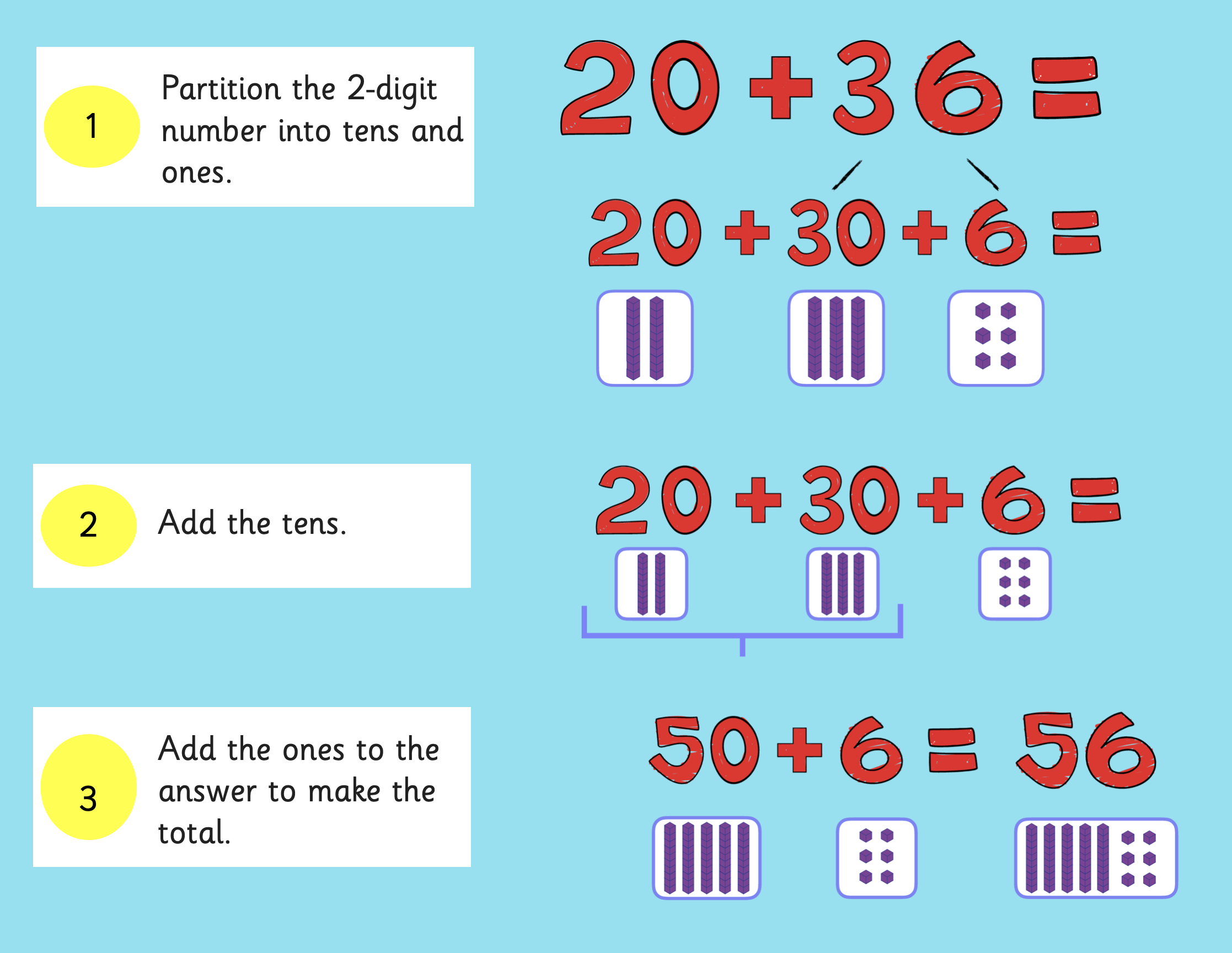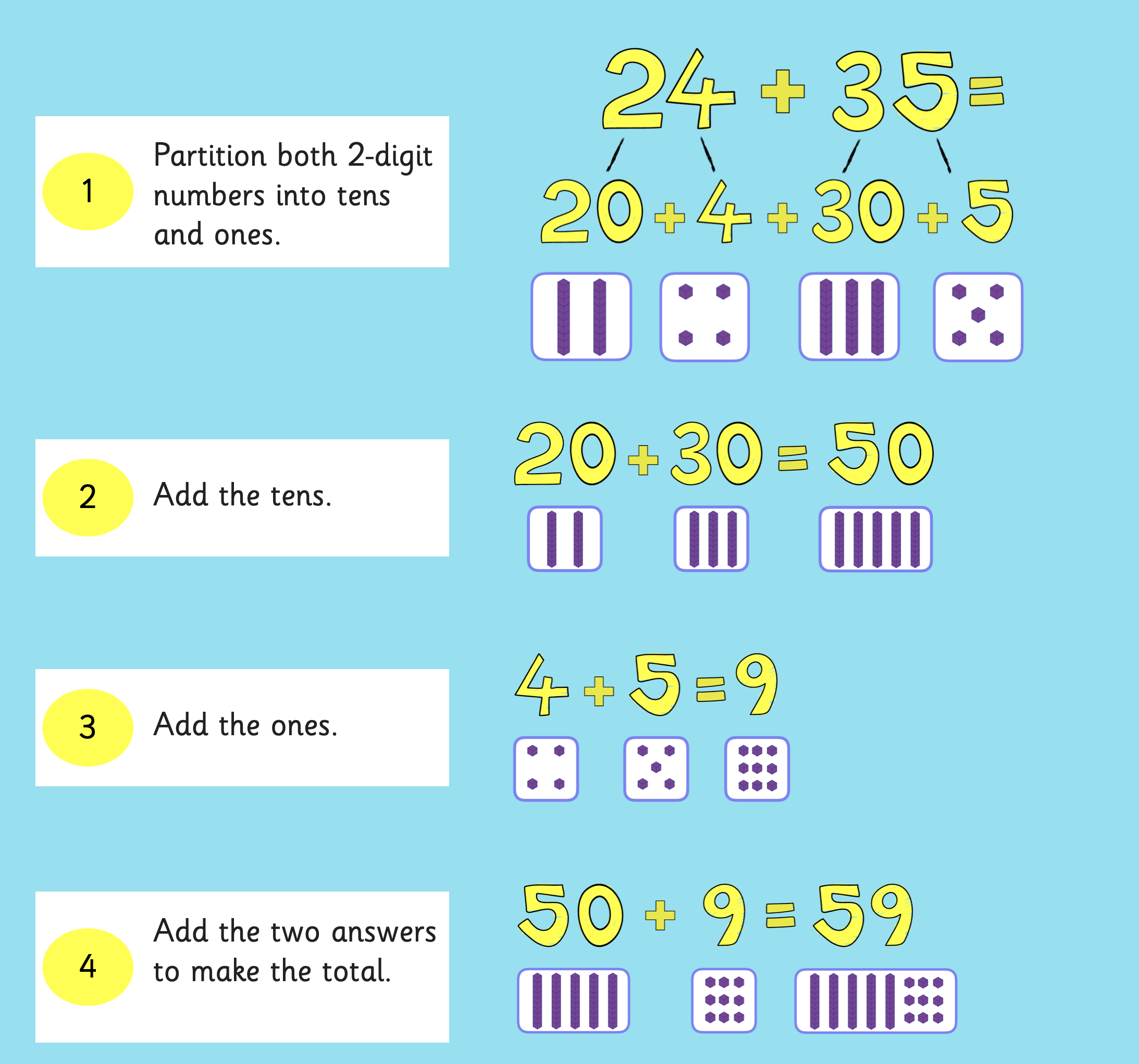Similarly, the same model works well for for teaching progression in subtraction.

How to partition for subtraction

1. Practise partitioning 2-digit numbers;
2. Use partitioning to subtract a 2-digit number from a multiple of ten;
3. Use partitioning to subtract one 2-digit number from another without bridging ten;
4. Use partitioning to subtract one 2-digit number from another bridging ten.

## Why is partitioning important?

Partitioning is a skill that children use throughout the KS1 and KS2 Maths curriculum.

## At KS1, partitioning helps to reinforce children's understanding of number composition and place value and also helps children to find efficient ways to add and subtract.

At KS2, partitioning is key to efficient and accurate exchange in formal column addition and subtraction, and a solid understanding of partitioning will help children to solve increasingly complex problems in the areas of fractions, ratio and proportion.